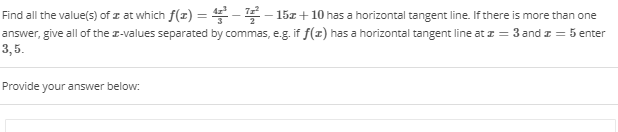# - 15 +10 has a horizontal tangent line. If there is Find all the value(s) of z at which f(z) 72. 15z + 10 has a horizontal tangent line. If there is more than one answer, give all of the z-values separated by commas, e.g. if f(z) has a horizontal tangent line atz 3 andz 5 enter 3,5. Provide your answer below:

Questionhelp_outlineImage Transcriptionclose- 15 +10 has a horizontal tangent line. If there is Find all the value(s) of z at which f(z) 72. 15z + 10 has a horizontal tangent line. If there is more than one answer, give all of the z-values separated by commas, e.g. if f(z) has a horizontal tangent line atz 3 andz 5 enter 3,5. Provide your answer below: fullscreen